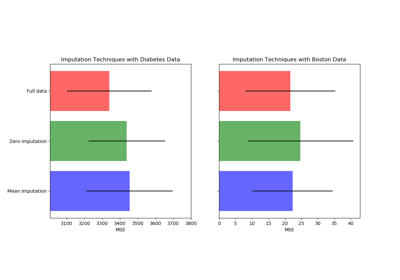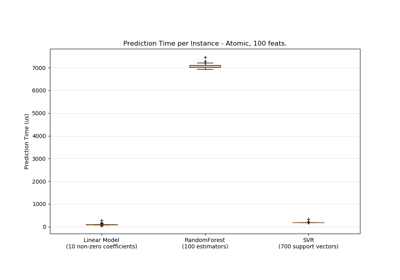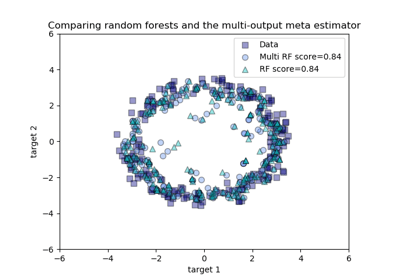/scikit-learn

3.2.4.3.2. sklearn.ensemble.RandomForestRegressor

class sklearn.ensemble.RandomForestRegressor(n_estimators=’warn’, criterion=’mse’, max_depth=None, min_samples_split=2, min_samples_leaf=1, min_weight_fraction_leaf=0.0, max_features=’auto’, max_leaf_nodes=None, min_impurity_decrease=0.0, min_impurity_split=None, bootstrap=True, oob_score=False, n_jobs=None, random_state=None, verbose=0, warm_start=False) [source]

A random forest regressor.

A random forest is a meta estimator that fits a number of classifying decision trees on various sub-samples of the dataset and uses averaging to improve the predictive accuracy and control over-fitting. The sub-sample size is always the same as the original input sample size but the samples are drawn with replacement if bootstrap=True (default).

Read more in the User Guide.

Parameters: n_estimators : integer, optional (default=10) The number of trees in the forest. Changed in version 0.20: The default value of n_estimators will change from 10 in version 0.20 to 100 in version 0.22. criterion : string, optional (default=”mse”) The function to measure the quality of a split. Supported criteria are “mse” for the mean squared error, which is equal to variance reduction as feature selection criterion, and “mae” for the mean absolute error. New in version 0.18: Mean Absolute Error (MAE) criterion. max_depth : integer or None, optional (default=None) The maximum depth of the tree. If None, then nodes are expanded until all leaves are pure or until all leaves contain less than min_samples_split samples. min_samples_split : int, float, optional (default=2) The minimum number of samples required to split an internal node: If int, then consider min_samples_split as the minimum number. If float, then min_samples_split is a fraction and ceil(min_samples_split * n_samples) are the minimum number of samples for each split. Changed in version 0.18: Added float values for fractions. min_samples_leaf : int, float, optional (default=1) The minimum number of samples required to be at a leaf node. A split point at any depth will only be considered if it leaves at least min_samples_leaf training samples in each of the left and right branches. This may have the effect of smoothing the model, especially in regression. If int, then consider min_samples_leaf as the minimum number. If float, then min_samples_leaf is a fraction and ceil(min_samples_leaf * n_samples) are the minimum number of samples for each node. Changed in version 0.18: Added float values for fractions. min_weight_fraction_leaf : float, optional (default=0.) The minimum weighted fraction of the sum total of weights (of all the input samples) required to be at a leaf node. Samples have equal weight when sample_weight is not provided. max_features : int, float, string or None, optional (default=”auto”) The number of features to consider when looking for the best split: If int, then consider max_features features at each split. If float, then max_features is a fraction and int(max_features * n_features) features are considered at each split. If “auto”, then max_features=n_features. If “sqrt”, then max_features=sqrt(n_features). If “log2”, then max_features=log2(n_features). If None, then max_features=n_features. Note: the search for a split does not stop until at least one valid partition of the node samples is found, even if it requires to effectively inspect more than max_features features. max_leaf_nodes : int or None, optional (default=None) Grow trees with max_leaf_nodes in best-first fashion. Best nodes are defined as relative reduction in impurity. If None then unlimited number of leaf nodes. min_impurity_decrease : float, optional (default=0.) A node will be split if this split induces a decrease of the impurity greater than or equal to this value. The weighted impurity decrease equation is the following: N_t / N * (impurity - N_t_R / N_t * right_impurity - N_t_L / N_t * left_impurity) where N is the total number of samples, N_t is the number of samples at the current node, N_t_L is the number of samples in the left child, and N_t_R is the number of samples in the right child. N, N_t, N_t_R and N_t_L all refer to the weighted sum, if sample_weight is passed. New in version 0.19. min_impurity_split : float, Threshold for early stopping in tree growth. A node will split if its impurity is above the threshold, otherwise it is a leaf. Deprecated since version 0.19: min_impurity_split has been deprecated in favor of min_impurity_decrease in 0.19 and will be removed in 0.21. Use min_impurity_decrease instead. bootstrap : boolean, optional (default=True) Whether bootstrap samples are used when building trees. oob_score : bool, optional (default=False) whether to use out-of-bag samples to estimate the R^2 on unseen data. n_jobs : int or None, optional (default=None) The number of jobs to run in parallel for both fit and predict. None` means 1 unless in a joblib.parallel_backend context. -1 means using all processors. See Glossary for more details. random_state : int, RandomState instance or None, optional (default=None) If int, random_state is the seed used by the random number generator; If RandomState instance, random_state is the random number generator; If None, the random number generator is the RandomState instance used by np.random. verbose : int, optional (default=0) Controls the verbosity when fitting and predicting. warm_start : bool, optional (default=False) When set to True, reuse the solution of the previous call to fit and add more estimators to the ensemble, otherwise, just fit a whole new forest. See the Glossary. estimators_ : list of DecisionTreeRegressor The collection of fitted sub-estimators. feature_importances_ : array of shape = [n_features] Return the feature importances (the higher, the more important the feature). n_features_ : int The number of features when fit is performed. n_outputs_ : int The number of outputs when fit is performed. oob_score_ : float Score of the training dataset obtained using an out-of-bag estimate. oob_prediction_ : array of shape = [n_samples] Prediction computed with out-of-bag estimate on the training set.

DecisionTreeRegressor, ExtraTreesRegressor

Notes

The default values for the parameters controlling the size of the trees (e.g. max_depth, min_samples_leaf, etc.) lead to fully grown and unpruned trees which can potentially be very large on some data sets. To reduce memory consumption, the complexity and size of the trees should be controlled by setting those parameter values.

The features are always randomly permuted at each split. Therefore, the best found split may vary, even with the same training data, max_features=n_features and bootstrap=False, if the improvement of the criterion is identical for several splits enumerated during the search of the best split. To obtain a deterministic behaviour during fitting, random_state has to be fixed.

The default value max_features="auto" uses n_features rather than n_features / 3. The latter was originally suggested in , whereas the former was more recently justified empirically in .

References

  Breiman, “Random Forests”, Machine Learning, 45(1), 5-32, 2001.
  P. Geurts, D. Ernst., and L. Wehenkel, “Extremely randomized trees”, Machine Learning, 63(1), 3-42, 2006.

Examples

>>> from sklearn.ensemble import RandomForestRegressor
>>> from sklearn.datasets import make_regression
>>>
>>> X, y = make_regression(n_features=4, n_informative=2,
...                        random_state=0, shuffle=False)
>>> regr = RandomForestRegressor(max_depth=2, random_state=0,
...                              n_estimators=100)
>>> regr.fit(X, y)
RandomForestRegressor(bootstrap=True, criterion='mse', max_depth=2,
max_features='auto', max_leaf_nodes=None,
min_impurity_decrease=0.0, min_impurity_split=None,
min_samples_leaf=1, min_samples_split=2,
min_weight_fraction_leaf=0.0, n_estimators=100, n_jobs=None,
oob_score=False, random_state=0, verbose=0, warm_start=False)
>>> print(regr.feature_importances_)
[0.18146984 0.81473937 0.00145312 0.00233767]
>>> print(regr.predict([[0, 0, 0, 0]]))
[-8.32987858]

Methods

 apply(X) Apply trees in the forest to X, return leaf indices. decision_path(X) Return the decision path in the forest fit(X, y[, sample_weight]) Build a forest of trees from the training set (X, y). get_params([deep]) Get parameters for this estimator. predict(X) Predict regression target for X. score(X, y[, sample_weight]) Returns the coefficient of determination R^2 of the prediction. set_params(**params) Set the parameters of this estimator.
__init__(n_estimators=’warn’, criterion=’mse’, max_depth=None, min_samples_split=2, min_samples_leaf=1, min_weight_fraction_leaf=0.0, max_features=’auto’, max_leaf_nodes=None, min_impurity_decrease=0.0, min_impurity_split=None, bootstrap=True, oob_score=False, n_jobs=None, random_state=None, verbose=0, warm_start=False) [source]
apply(X) [source]

Apply trees in the forest to X, return leaf indices.

Parameters: X : array-like or sparse matrix, shape = [n_samples, n_features] The input samples. Internally, its dtype will be converted to dtype=np.float32. If a sparse matrix is provided, it will be converted into a sparse csr_matrix. X_leaves : array_like, shape = [n_samples, n_estimators] For each datapoint x in X and for each tree in the forest, return the index of the leaf x ends up in.
decision_path(X) [source]

Return the decision path in the forest

New in version 0.18.

Parameters: X : array-like or sparse matrix, shape = [n_samples, n_features] The input samples. Internally, its dtype will be converted to dtype=np.float32. If a sparse matrix is provided, it will be converted into a sparse csr_matrix. indicator : sparse csr array, shape = [n_samples, n_nodes] Return a node indicator matrix where non zero elements indicates that the samples goes through the nodes. n_nodes_ptr : array of size (n_estimators + 1, ) The columns from indicator[n_nodes_ptr[i]:n_nodes_ptr[i+1]] gives the indicator value for the i-th estimator.
feature_importances_
Return the feature importances (the higher, the more important the
feature).
Returns: feature_importances_ : array, shape = [n_features]
fit(X, y, sample_weight=None) [source]

Build a forest of trees from the training set (X, y).

Parameters: X : array-like or sparse matrix of shape = [n_samples, n_features] The training input samples. Internally, its dtype will be converted to dtype=np.float32. If a sparse matrix is provided, it will be converted into a sparse csc_matrix. y : array-like, shape = [n_samples] or [n_samples, n_outputs] The target values (class labels in classification, real numbers in regression). sample_weight : array-like, shape = [n_samples] or None Sample weights. If None, then samples are equally weighted. Splits that would create child nodes with net zero or negative weight are ignored while searching for a split in each node. In the case of classification, splits are also ignored if they would result in any single class carrying a negative weight in either child node. self : object
get_params(deep=True) [source]

Get parameters for this estimator.

Parameters: deep : boolean, optional If True, will return the parameters for this estimator and contained subobjects that are estimators. params : mapping of string to any Parameter names mapped to their values.
predict(X) [source]

Predict regression target for X.

The predicted regression target of an input sample is computed as the mean predicted regression targets of the trees in the forest.

Parameters: X : array-like or sparse matrix of shape = [n_samples, n_features] The input samples. Internally, its dtype will be converted to dtype=np.float32. If a sparse matrix is provided, it will be converted into a sparse csr_matrix. y : array of shape = [n_samples] or [n_samples, n_outputs] The predicted values.
score(X, y, sample_weight=None) [source]

Returns the coefficient of determination R^2 of the prediction.

The coefficient R^2 is defined as (1 - u/v), where u is the residual sum of squares ((y_true - y_pred) ** 2).sum() and v is the total sum of squares ((y_true - y_true.mean()) ** 2).sum(). The best possible score is 1.0 and it can be negative (because the model can be arbitrarily worse). A constant model that always predicts the expected value of y, disregarding the input features, would get a R^2 score of 0.0.

Parameters: X : array-like, shape = (n_samples, n_features) Test samples. For some estimators this may be a precomputed kernel matrix instead, shape = (n_samples, n_samples_fitted], where n_samples_fitted is the number of samples used in the fitting for the estimator. y : array-like, shape = (n_samples) or (n_samples, n_outputs) True values for X. sample_weight : array-like, shape = [n_samples], optional Sample weights. score : float R^2 of self.predict(X) wrt. y.
set_params(**params) [source]

Set the parameters of this estimator.

The method works on simple estimators as well as on nested objects (such as pipelines). The latter have parameters of the form <component>__<parameter> so that it’s possible to update each component of a nested object.

Returns: self

3.2.4.3.2.1. Examples using sklearn.ensemble.RandomForestRegressorImputing missing values before building an estimatorPrediction LatencyComparing random forests and the multi-output meta estimator

© 2007–2018 The scikit-learn developers